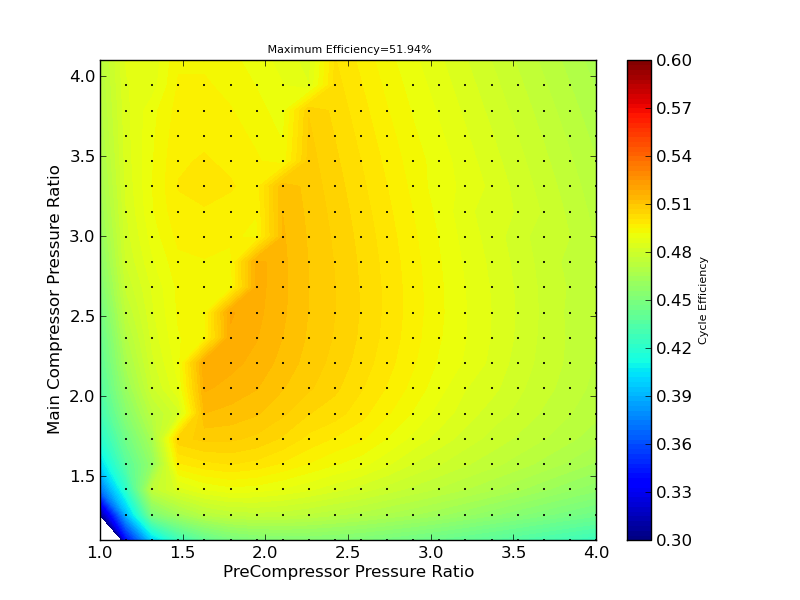Supercritical Carbon Dioxide Power Cycle Design Space Explorer

## Notice: I've enhanced the Real Fluid Heat Engine Cycle Analysis Code since this web page was created to generate more accurate results, but have not yet had the time to update this web page to use that new solver. Also, the number of parameters explored in the dataset presented below was simplified. Adding all relevant parameters to a brute force study results in an unwieldy computational task and I don't believe that a brute force design space exploration process is always the best approach. Therefor, this web page is being maintained mostly as a historical reference. My doctoral dissertation, A Study of Power Cycles Using Supercritical Carbon Dioxide as the Working Fluid, utilizes a more intelligent approach to explore portions of the design space by optimizing select parameters using a hybrid stochastic and gradient based approach. Please see Chapter 2 of this document, or install the Real Fluid Heat Engine Cycle Analysis Code on your computer to run the design study of your choice.

Below are the results of the current design space exploration. Details regarding the methodology used and the layouts considered can be found in the document Mapping the Design Space of a Recuperated, Recompression, Precompression Supercritical Carbon Dioxide Power Cycle with Intercooling, Improved Regeneration, and Reheat. You can use the selectors to change the figures that are displayed and explore the cycle.

Notes:

• Sensitivity Contour Plot horizontal axis and vertical axis cannot be the same selection. If they are selected to be the same, an error message will be displayed for the Sensitivity Contour Plot.
• Sensitivity Contour Plot contour level cannot be the same selection as the horizontal or vertical axis. If they are selected to be the same, the contour level will revert to "Maximum Cycle Efficiency".
• Sensitivity Line Plot horizontal axis and vertical axis cannot be the same selection. If both are selected to be the same, the vertical axis will revert to "Maximum Cycle Efficiency".
• ReCompressor pressure ratio (which is not yet plottable) will be close to the main compressor pressure ratio (the difference is due to heat exchanger pressure ratios, which are close to 1). Plotting the ReCompressor pressure ratio and other dependent variables may be a feature soon.

 Sensitivity Plots and Cycle Plot Sensitivity Plots Horizontal Axis Vertical Axis Contour Level Vertical Axis Independent Variable Value Selected(selection ignored if variable is used for a sensitivity plot axis) Contour and Line Plots Contour Plot Plot Value for Maximum Efficiency Contour Plot Line Plot Dataset 20,155,392 permutations - All Parameters - Coarse Exploration1,800,000 permutations - Fixed Component Efficiencies and Max/Min Temp, Other Parameters Refined PreCompressor Pressure Ratio Value for Maximum Efficiency 1.0 1.15789473684 1.31578947368 1.47368421053 1.63157894737 1.78947368421 1.94736842105 2.10526315789 2.26315789474 2.42105263158 2.57894736842 2.73684210526 2.89473684211 3.05263157895 3.21052631579 3.36842105263 3.52631578947 3.68421052632 3.84210526316 4.0 Main Compressor Pressure Ratio Value for Maximum Efficiency 1.1 1.25789473684 1.41578947368 1.57368421053 1.73157894737 1.88947368421 2.04736842105 2.20526315789 2.36315789474 2.52105263158 2.67894736842 2.83684210526 2.99473684211 3.15263157895 3.31052631579 3.46842105263 3.62631578947 3.78421052632 3.94210526316 4.1 Recompression Fraction Value for Maximum Efficiency 0.0 0.0707857142857 0.141571428571 0.212357142857 0.283142857143 0.353928571429 0.424714285714 0.4955 0.566285714286 0.637071428571 0.707857142857 0.778642857143 0.849428571429 0.920214285714 0.991 Low Temperature Recuperator Main Fraction High Pressure Component Mass Fraction Value for Maximum Efficiency 0.001 0.0717142857143 0.142428571429 0.213142857143 0.283857142857 0.354571428571 0.425285714286 0.496 0.566714285714 0.637428571429 0.708142857143 0.778857142857 0.849571428571 0.920285714286 0.991 Main Compressor Inlet Pressure [Pa] Value for Maximum Efficiency 6000000.0 6210526.31579 6421052.63158 6631578.94737 6842105.26316 7052631.57895 7263157.89474 7473684.21053 7684210.52632 7894736.84211 8105263.15789 8315789.47368 8526315.78947 8736842.10526 8947368.42105 9157894.73684 9368421.05263 9578947.36842 9789473.68421 10000000.0 Maximum Temperature [K] Value for Maximum Efficiency 923.0 Minimum Temperature [K] Value for Maximum Efficiency 320.0 Main Compressor Isentropic Efficiency Value for Maximum Efficiency 0.85 PreCompressor Isentropic Efficiency Value for Maximum Efficiency 0.875 ReCompressor Isentropic Efficiency Value for Maximum Efficiency 0.875 Power Turbine Isentropic Efficiency Value for Maximum Efficiency 0.93 Main/Re/Pre Compressor Turbine Isentropic Efficiency Value for Maximum Efficiency 0.89 Heat Exchanger Pressure Drop [Pa/K] Value for Maximum Efficiency 500.0 Sensitivity Plot Dependent Variable Plot Value Contour Plot Line Plot Maximum Cycle Efficiency Cycle Plot Quantity Horizontal Axis Vertical Axis Contour Level None (loads quicker) Temperature Pressure Enthalpy Entropy Density CompressibilityFactor cp gamma
Open a New WindowSensitivity Contour PlotSensitivity Line PlotCycle PlotNotes: This Plot will take about 15 seconds to display. Please be patient!You can change the Cycle Plot Contour Level to None in order to speed up loading.Cycle Layout Notes: State points match those used in the Cycle Plot. If the PreCompressor outlet temperature is less than or equal to Main Compressor outlet temperature, the low temperature recuperators will not be used and points 3, 12, and 15 will not be present in the Cycle Plot. If the PreCompressor Pressure Ratio is set to 1 (no PreCompressor), no medium and low temperature recuperators will not be used and points 3, 11, 12, and 15 will not be present in the Cycle Plot Currently the PreCompressor inlet temperature is equal to the Main Compressor Inlet temperature, and cannot yet be set to a higher temperature. "HOT" indicates a heat source, and "COLD" indicates a cold sink such as a cooling tower.©2007 - Andy Schroder